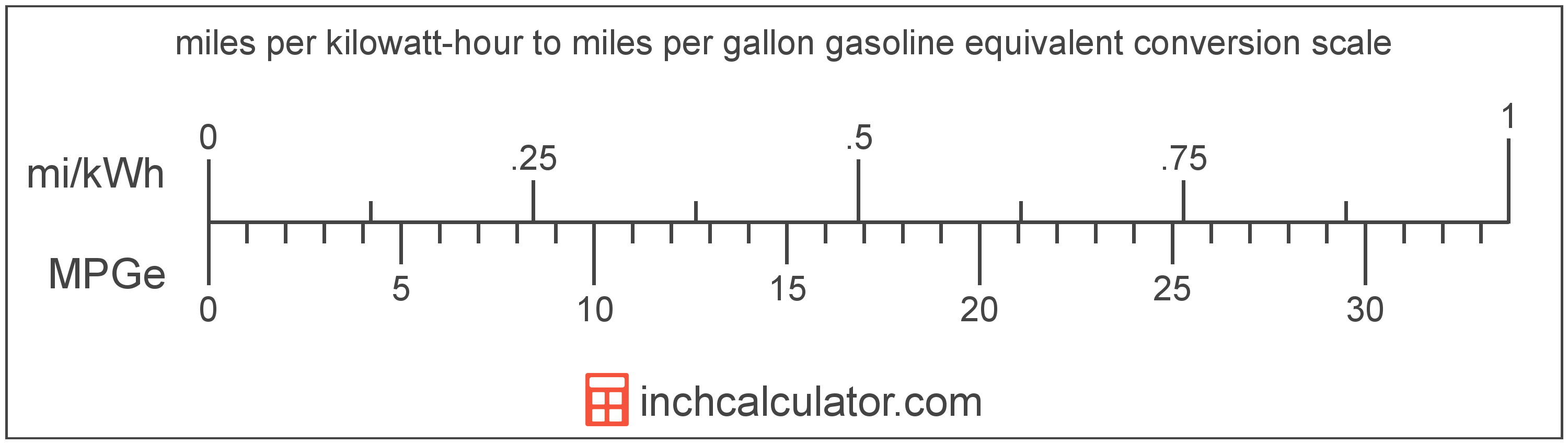# Miles Per Gallon Gasoline Equivalent to Miles Per Kilowatt-hour Conversion (MPGe to Mi/kWh)

Enter the electric car efficiency in miles per gallon gasoline equivalent below to get the value converted to miles per kilowatt-hour.

Results in Miles Per Kilowatt-hour:1 MPGe = 0.029669 mi/kWh
Do you want to convert mi/kWh to MPGe?

## How to Convert Miles Per Gallon Gasoline Equivalent to Miles Per Kilowatt-hourConvert miles per gallon gasoline equivalent to miles per kilowatt-hour with this simple formula:

mi/kWh = MPGe ÷ 33.705

Insert the MPGe efficiency measurement in the formula and then solve to find the result.

For example, let's convert 50 MPGe to mi/kWh:

50 MPGe = ( 50 ÷ 33.705 ) = 1.483459 mi/kWh

Miles per gallon gasoline equivalent and miles per kilowatt-hour are both units used to measure electric car efficiency. Keep reading to learn more about each unit of measure.

## Miles Per Gallon Gasoline Equivalent

Miles per gallon gasoline equivalent is a way of measuring the energy needed equivalent to a gallon of gasoline to travel a distance in miles.

Because electric vehicles do not consume gasoline, the EPA set an equivalent amount of energy in kilowatt-hours that is equal to one gallon of gasoline. According to the EPA, one gallon of gasoline is equal to 33.705 kilowatt-hours.

The mile per gallon gasoline equivalent is a US customary unit of electric car efficiency. Miles per gallon gasoline equivalent can be abbreviated as MPGe; for example, 1 mile per gallon gasoline equivalent can be written as 1 MPGe.

## Miles Per Kilowatt-hour

Miles per kilowatt-hour is a measure of electric vehicle efficiency equal to the distance traveled in miles consuming one kilowatt-hour of energy.

The mile per kilowatt-hour is a US customary unit of electric car efficiency. Miles per kilowatt-hour can be abbreviated as mi/kWh; for example, 1 mile per kilowatt-hour can be written as 1 mi/kWh.

In formal expressions, the slash, or solidus (/), is used to separate units used to indicate division in an expression.

## Mile Per Gallon Gasoline Equivalent to Mile Per Kilowatt-hour Conversion Table

Mile per gallon gasoline equivalent measurements converted to miles per kilowatt-hour
Miles Per Gallon Gasoline Equivalent Miles Per Kilowatt-hour
1 MPGe 0.029669 mi/kWh
2 MPGe 0.059338 mi/kWh
3 MPGe 0.089008 mi/kWh
4 MPGe 0.118677 mi/kWh
5 MPGe 0.148346 mi/kWh
6 MPGe 0.178015 mi/kWh
7 MPGe 0.207684 mi/kWh
8 MPGe 0.237354 mi/kWh
9 MPGe 0.267023 mi/kWh
10 MPGe 0.296692 mi/kWh
11 MPGe 0.326361 mi/kWh
12 MPGe 0.35603 mi/kWh
13 MPGe 0.385699 mi/kWh
14 MPGe 0.415369 mi/kWh
15 MPGe 0.445038 mi/kWh
16 MPGe 0.474707 mi/kWh
17 MPGe 0.504376 mi/kWh
18 MPGe 0.534045 mi/kWh
19 MPGe 0.563715 mi/kWh
20 MPGe 0.593384 mi/kWh
21 MPGe 0.623053 mi/kWh
22 MPGe 0.652722 mi/kWh
23 MPGe 0.682391 mi/kWh
24 MPGe 0.712061 mi/kWh
25 MPGe 0.74173 mi/kWh
26 MPGe 0.771399 mi/kWh
27 MPGe 0.801068 mi/kWh
28 MPGe 0.830737 mi/kWh
29 MPGe 0.860406 mi/kWh
30 MPGe 0.890076 mi/kWh
31 MPGe 0.919745 mi/kWh
32 MPGe 0.949414 mi/kWh
33 MPGe 0.979083 mi/kWh
34 MPGe 1.0088 mi/kWh
35 MPGe 1.0384 mi/kWh
36 MPGe 1.0681 mi/kWh
37 MPGe 1.0978 mi/kWh
38 MPGe 1.1274 mi/kWh
39 MPGe 1.1571 mi/kWh
40 MPGe 1.1868 mi/kWh

## References

1. United States Environmental Protection Agency, Technology, https://www3.epa.gov/otaq/gvg/learn-more-technology.htm